# Complex-Valued Neurocomputing and Singular Points

AUTHORSTohru Nitta 1 , *

1 Human Informatics Research Institute, National Institute of Advanced Industrial Science and Technology (AIST), Tsukuba, Japan

How to Cite: Nitta T. Complex-Valued Neurocomputing and Singular Points. Arch Neurosci. 2015;2(4):e27461.
doi: 10.5812/archneurosci.27461.

ARTICLE INFORMATION

Archives of Neuroscience: 2 (4); e27461
Published Online: October 10, 2015
Article Type: Review Article
Accepted: March 3, 2015Crossmark
CHECKING

### Abstract

#### Context:

Recently, the singular points of neural networks have attracted attention from the artificial intelligence community, and their interesting properties have been demonstrated. The objective of this study is to provide an overview of studies on the singularities of complex-valued neural networks.

#### Evidence Acquisition:

This review is based on the relevant literature on complex-valued neural networks and singular points.

#### Results:

Review of the studies and available literature on the subject area shows that the singular points of complex-valued neural networks have negative effects on learning, as do those of real-valued neural networks. However, the nature of the singular points in complex-valued neural networks is superior in quality, and the methods for improving the learning performance have been proposed.

#### Conclusions:

A complex-valued neural network could be a promising learning method from the viewpoint of a singularity.

### 1. Context

An artificial neural network is a machine learning model and has recently attracted attention owing to its human-like intelligence such as learning and generalization (1).

A complex-valued neural network extends (real-valued) parameters (weights and threshold values) in a real-valued neural network to complex numbers. It is advantageous because the good-natured behavior of a complex number to rotate is assured. Thus, it is suitable for the information processing of complex-valued data and two-dimensional data and has been applied to various fields such as communications, image processing, biological information processing, land-mine detection, wind prediction, and independent component analysis (ICA) (2). Recently, a complex-valued firing-rate model was presented (3), which is an attempt to implement a neural network of an actual brain with complex numbers.

Generally, a hierarchical structure causes singular points. For example, consider a three-layered real-valued neural network. If a weight between a hidden neuron and an output neuron is equal to zero, then no value of the weight vector between the hidden neuron and the input neurons affects the output value of the real-valued neural network. Then, the weight vector is called an unidentifiable parameter, and is a singular point. It has been shown that singular points affect the learning dynamics of learning models and that they can cause a standstill in learning (4-6).

This paper reviews the current state of studies on the singularities of complex-valued neural networks.

### 2. Evidence Acquisition

This review is based on the relevant literature on complex-valued neural networks and singular points. Although the results in the literature are obtained by mathematical analyses and computer simulations, the use of mathematical expressions has been avoided as much as possible in this paper for the sake of simplicity.

### 3. Results

#### 3.1. A Single Complex-Valued Neuron

The properties of the singular points of a complex-valued neuron constituting a complex-valued neural network have been described (7-9). There are two types of complex-valued neurons: a complex-valued neuron whose parameters (weight and threshold) are expressed with orthogonal coordinates (e.g., x + iy) and a complex-valued neuron whose parameters are expressed with polar coordinates (e.g., r exp[iθ]), called a polar-variable complex-valued neuron. It is trivial that the former complex-valued neuron model does not have any singular points. However, the polar-variable complex-valued neuron has many singular points. The singular points bring about various properties in the polar-variable complex-valued neuron model.

Firstly, the parameters of a polar-variable complex-valued neuron are unidentifiable. That is, if an amplitude parameter r is equal to zero, then r exp[iθ] z = 0 holds for any input signal z, and no value of the phase parameter θ affects the output value of a complex-valued neuron. Thus, we cannot identify the value of θ by learning. Therefore, it is verified that θ is an unidentifiable parameter, and a polar-variable complex-valued neuron has an unidentifiable nature.

Secondly, a plateau phenomenon could occur during learning when using the steepest descent method with a squared error. That is a learning period in which the learning error cannot be reduced occurs during learning, where a “learning period” and “learning error” are usually used as proper names in the field of neural networks. Thus, it has been experimentally suggested that unidentifiable parameters (singular points) degrade the learning speed (7-9).

Finally, it was suggested experimentally that the steepest gradient descent method with an amplitude-phase error (10) and the complex-valued natural gradient descent method (11) are effective for improving the learning performance.

#### 3.2. Three-Layered Complex-Valued Neural Network

Since there have been no studies on three-layered complex-valued neural networks consisting of polar-variable complex-valued neurons, three-layered complex-valued neural networks consisting of only complex-valued neurons represented by orthogonal coordinates are discussed in this section.

#### 3.2.1. Local Minima of a Three-Layered Complex-Valued Neural Network

Consider a three-layered complex-valued neural network with L input neurons, H hidden neurons, and one output neuron. The hierarchical structure of the three-layered complex-valued neural network yields three types of redundancies (Figure 1) (12). (a) In the upper part of Figure 1, the hidden neuron j of the complex-valued neural network never influences the output neuron because the weight vj between the hidden neuron j and the output neuron is equal to zero. Thus, we can remove the hidden neuron j. (b) In the middle part of Figure 1, the output of the hidden neuron j of the complex-valued neural network is only a constant k because the weight vector between the input neurons and the hidden neuron j is equal to zero: wj = 0; then, we can remove the hidden neuron j and replace the threshold of the output neuron v0with v0 + k. (c) In the lower part of Figure 1, we can remove the hidden neuron j2 and replace the weight vj1 between the hidden neuron j1 and the output neuron with vj1 + qvj2, where q = -1, 1, -i, or i, and vj2 is the weight between the hidden neuron j2 and the output neuron because w~j1=qw~j2, where w~j1 is the vector that consists of the weight vector between the input neurons and the hidden neuron j1 and the threshold of the hidden neuron j1, and w~j2 is the vector that consists of the weight vector between the input neurons and the hidden neuron j2 and the threshold of the hidden neuron j2.

Figure 1. Three Types of Redundancies of a Three-Layered Complex-Valued Neural Network (© 2013, Elsevier. Used With Permission), % Not Yet Obtained

The three types of redundancies described above yield the critical point at which the learning error is unchanged (12). There are three types of critical points: a local minimum, local maximum, and saddle point, which can be identified using the Hessian, as is well known. In the case of real-valued neural networks, the redundancies corresponding to redundancies (a) and (b) of the complex-valued neural network described above inevitably yield saddle points, and the redundancy corresponding to redundancy (c) of the complex-valued neural network described above yields saddle points or local minima according to the conditions (13). Fukumizu and Amari (13) confirmed that the local minima caused 50,000 plateaus using computer simulations, which had a strong negative influence on learning. It was proved that most of local minima that Fukumizu and Amari (13) discovered could be resolved by extending the real-valued neural network to complex numbers; most of the critical points caused by the hierarchical structure of the complex-valued neural network are saddle points, which is a prominent property of the complex-valued neural network (12). Note that such local minima are only those caused by the hierarchical structures of the complex-valued neural network; Local minima of the other types might exist in the complex-valued neural network. Recently, it has been shown that there exists a reducibility of another type (called exceptional reducibility) (14). It is important to clarify how the exceptional reducibility is related to the local minima of complex-valued neural networks.

#### 3.2.2. Learning Dynamics of the Three-Layered Complex-Valued Neural Network in the Neighborhood of Singular Points

The linear combination structure in the updating rule for the learnable parameters of a complex-valued neural network increases the speed moving away from the singular points; the complex-valued neural network could not be easily influenced by the singular points (15).

Consider a 1-1-1 complex-valued neural network (one input neuron, one hidden neuron, and one output neuron) and a 2-1-2 real-valued neural network (two input neurons, one hidden neuron, and two output neurons) for the sake of simplicity. The number of learnable parameters (weights and thresholds) of the 2-1-2 real-valued neural network is seven, which is almost equal to the number of learnable parameters eight of the 1-1-1 complex-valued neural network. Thus, the comparison of the learning dynamics using those neural networks is fair.

The average learning dynamics are investigated, assuming that the standard gradient learning method is used. The following are the explanatory equations of the learning dynamics of the two neural networks: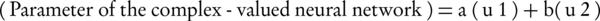(1)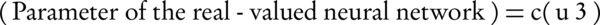(2)

Here, ∅ is a real-valued activation function. A split-type activation function ∅(x) + i∅(y) is used for the complex-valued neuron, where i = √-1 and z = x + iy is the net input into the complex-valued neuron. For example, if a = b = c and u1 = u2 = u3, then Δ (Parameter of the complex-valued neural network) = 2a∅(u1) = 2∆ (Parameter of the real-valued neural network) holds. Moreover, Δ (Parameter of the complex neural network) cannot be easily equal to zero because a∅(u1) is not necessarily equal to zero, even if one term in b∅(u2) is almost equal to zero. Thus, we can assume that the speed of the complex-valued neural network moving away from the singularity is faster than that of the real-valued neural network.

#### 3.2.3. Construction of Complex-Valued Neural Networks That do Not Have Critical Points Based on a Hierarchical Structure

It has been shown that the decomposition of high-dimensional neural networks into low-dimensional neural networks equivalent to the original neural networks yields neural networks that have no critical points based on the hierarchical structure (16). As for the case of complex-valued neural networks, a 2-2-2 three-layered complex-valued neural network can be constructed from a 1-1-1 three-layered quaternionic neural network. Such a complex-valued neural network does not comparatively suffer from negative effects caused by singular points during learning because it has no critical points based on a hierarchical structure.

The practical implementation of the 2-2-2 complex-valued neural network having no critical points based on a hierarchical structure is as follows.

1. Consider a 1-1-1 quaternionic neural network (called NET 1 here). Let the weight between the input neuron and the hidden neuron be A = a + ib +jc +kd ϵ Q and the weight between a hidden neuron and an output neuron be B = α + iB +jγ +kδ ϵ Q, where Q represents the set of quaternions. The quaternion is a four-dimensional number and was invented by W. R. Hamilton in 1843 (17). Let C = p + iq + jr +ks ϵ Q denote the threshold of the hidden neuron and D = µ + iv + jρ +kσ ϵ Q represent the threshold of the output neuron. For a technical reason, we assume that D = 0. The activation functions are defined by the following equations: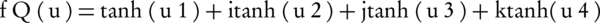(3)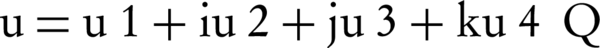(4)

for the hidden neuron, and:(5)

for the output neuron. For the sake of simplicity, we omitted the additional assumptions (see (16) for the details).

2. Create a 2-2-2 complex-valued neural network (called NET 2 here) by decomposing NET 1 described above, where a quaternion is decomposed into two complex numbers. That is, the quaternion A = a + ib + jc + kd ϵ Q representing the quaternionic weight between the input neuron and the hidden neuron is decomposed into the two complex numbers a′ = a +ib ϵ C and c′ = c + id ϵ C, where C is the set of complex numbers. Here, we used the Cayley-Dickson notation: the weight A between the input neuron and the hidden neuron of NET 1 can be written using Cayley-Dickson notation as follows: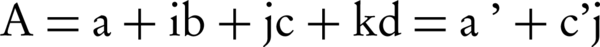(6)

where a’ = a + ib ϵ C and c’ = c + id ϵ C.

Similarly, the quaternion B = α + iβ + jγ +kδ ϵ Q representing the quaternionic weight between the hidden neuron and the output neuron is decomposed into the two complex numbers α′ = α +iβ ϵ C and dγ′ = γ + iδ ϵ C. The quaternion C = p + iq + jr + ks ϵ Q representing the quaternionic threshold of the hidden neuron is decomposed into two complex numbers p′ = p + iq ϵ C and r′ = r + is ϵ C. We use the activation function defined by the following equations for NET 2: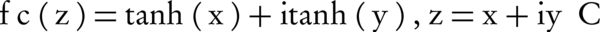(7)

for the hidden neuron, and(8)

for the output neuron.

NET 2 has no critical points based on a hierarchical structure (as a complex-valued neural network). See the literature (16) for the proof.

### 4. Conclusions

The author feels that the research results presented in this paper are probably only scratching the surface of the characteristics of the singularities of complex-valued neural networks. We believe that the results reviewed in this paper will be a clue to analyze the various types of singular points and to provide more excellent complex-valued neural networks. The problem of whether or not the complex-valued neural network has fewer local minima than the real-valued neural network remains unsolved. This is an important but difficult problem. In Section 3.2.3, a method for constructing a neural network that has no critical points based on a hierarchical structure is described. This is a theoretical result, and its empirical study is desired in future work.

### References

• 1.

Bengio Y. Learning deep architectures for AI. 2009; : 1 -127

• 2.

Nitta T. Complex-Valued Neural Networks: Utilizing High-Dimensional Parameters. 2009; : 504

• 3.

Schaffer ES, Ostojic S, Abbott LF. A complex-valued firing-rate model that approximates the dynamics of spiking networks. PLoS Comput Biol. 2013; 9 (10)[DOI][PubMed]

• 4.

Amari S, Park H, Ozeki T. Singularities affect dynamics of learning in neuromanifolds. Neural Comput. 2006; 18 (5) : 1007 -65 [DOI][PubMed]

• 5.

Wei H, Zhang J, Cousseau F, Ozeki T, Amari S. Dynamics of learning near singularities in layered networks. Neural Comput. 2008; 20 (3) : 813 -43 [DOI][PubMed]

• 6.

Cousseau F, Ozeki T, Amari S. Dynamics of learning in multilayer perceptrons near singularities. IEEE Trans Neural Netw. 2008; 19 (8) : 1313 -28 [DOI][PubMed]

• 7.

Nitta T. On the Singularity of a Single Complex-Valued Neuron [in Japanese]. IEICE Trans Inf SystD. 2010; J93-D (8) : 1614 -21

• 8.

Nitta T. Plateau in a Polar Variable Complex-Valued Neuron. ICAART2014 the 6th International Conference on Agents and Artificial Intelligence. : 526 -31

• 9.

Nitta T. Learning dynamics of a single polar variable complex-valued neuron. Neural Comput. 2015; 27 (5) : 1120 -41 [DOI][PubMed]

• 10.

Savitha R, Suresh S, Sundararajan N. Projection-based fast learning fully complex-valued relaxation neural network. IEEE Trans Neural Netw Learn Syst. 2013; 24 (4) : 529 -41 [DOI][PubMed]

• 11.

Nitta T. Natural Gradient Descent for Training Stochastic Complex-Valued Neural Networks. Int J Adv Comput Sci Appl. 2014; 5 (7) : 193 -8 [DOI]

• 12.

Nitta T. Local minima in hierarchical structures of complex-valued neural networks. Neural Netw. 2013; 43 : 1 -7 [DOI][PubMed]

• 13.

Fukumizu K, Amari S. Local minima and plateaus in hierarchical structures of multilayer perceptrons. Neural Netw. 2000; 13 (3) : 317 -27 [DOI]

• 14.

Kobayashi M. Exceptional reducibility of complex-valued neural networks. IEEE Trans Neural Netw. 2010; 21 (7) : 1060 -72 [DOI][PubMed]

• 15.

Nitta T. Learning Dynamics of the Complex-Valued Neural Network in the Neighborhood of Singular Points. J Comput Commun. 2014; 2 (1) : 27 -32 [DOI]

• 16.

Nitta T. Construction of Neural Networks that Do Not Have Critical Points Based on Hierarchical Structure. Int J Adv Comput Sci Appl. 2013; 4 (9) : 68 -73 [DOI]

• 17.

Gürlebeck K, Habetha K, Sprößig W. Holomorphic functions in the plane and n-dimensional space. 2008;

Copyright © 2015, Tehran University of Medical Sciences. This is an open-access article distributed under the terms of the Creative Commons Attribution-NonCommercial 4.0 International License (http://creativecommons.org/licenses/by-nc/4.0/) which permits copy and redistribute the material just in noncommercial usages, provided the original work is properly cited.